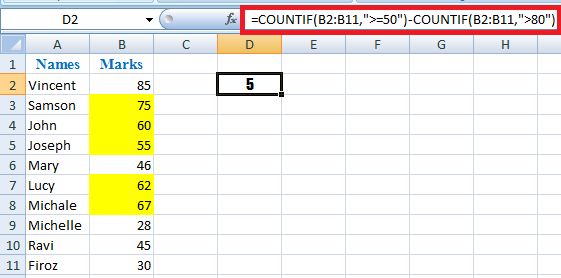# Excel Formula to Count Cells between Two Numbers

Count Cells between Two Numbers using Excel COUNTIF Formula

What is it:

If you are familiar with Microsoft Excel you would know that COUNTIF is the function use to count the number of cells that contains value.

In this post we are going to show you a formula to count the number of cell that contains value between two numbers in a particular column or a range of cells by using the combination of two COUNIF functions in the same formula.

Why is it important:

This formula is very helpful when you don’t want the count of entire cells in a column or a particular range of cells but only the count cells between two numbers.

For eg: You have a list of 10 students with their marks for a particular subject and you want to know out of 10 students, the count of students who scored 50 to 80.#### How to Count Cells between Two Numbers using COUNTIF formula:

I’ll explain you the formula with the same example above, its pretty simple; just a small subtract logic we are applying with COUNTIF function.

• First it counts all the numbers above 50. In this example we have 85, 75, 60, 55, 46, 62, 67, 28, 45, 30 that means 6 numbers are above 50. That’s what the first section of the formula does.

=COUNTIF(B2:B11,”>=50″)

• Secondly we are counting all the numbers above 80 and subtracting it from the first count.  In this example we have only 1 number above 80 ie 85.

COUNTIF(B2:B11,”>80″)

In short 6-1=5, and that’s what the result we are looking for, count of students who scored between 50 to 80.

So the complete formula will be like the below:

=COUNTIF(B2:B11,”>=50″)-COUNTIF(B2:B11,”>80″)

Instead of mentioning a specific range of cells if you want to count from entire column then your formula should be something like the below:

=COUNTIF(B:B,”>=50″)-COUNTIF(B:B,”>80″)

If you want to pick the starting and ending numbers/the between numbers from different cells instead of mentioning it directly in the formula (In this example 50 and 80), you can mention that cell value in the formula like below:

=COUNTIF(B2:B11,”>” &E1)-COUNTIF(B2:B11,”>”&F1)

So now you know how to count cells between two numbers in Excel using simple COUNTIF function.#### Anson Antony

Anson is a contributing author and founder at ASKEYGEEK.com. Learning anything new has always been his passion, ASKEYGEEK.com is an outcome of his passion for technology and business. He has got a decade of versatile experience in Business Process Outsourcing, Finance & Accounting, Information Technology, Operational Excellence & Business Intelligence. During the tenure, he had worked for organizations like Genpact, Hewlett Packard, M*Modal and Capgemini in various roles and responsibilities. Outside business and technology, he is a movie buff who spends hours together watching and learning Cinema and a Film Maker too!

## Over 608K+ Users Per Month

#### Weekly Tutorial